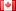# Revision history [back]

### Extension/coercion of finite rings & fields

Hi ... again harping on my theme of p-adics: here is a characteristic-p toy model of the problem I had in http://ask.sagemath.org/question/2309/solving-polynomial-equations-over-p-adic-fields. Will someone please tell how to do what this code is trying to do?!!

sage: K.<X> = GF(3^2,name='X'); # creates (residue) field which is GF(3)[X]

sage: R = IntegerModRing(3^2)[X]; # extends Z/p^2 by that same X to form quadratic extn - seemingly successfully

sage: print R(X+5); # I try to use X but it still treats it like an "alien" quantity in R ....

thanks!!2 improved stylefidbc2298 ●4 ●24 ●55

### Extension/coercion of finite rings & fields

Hi ...

again harping on my theme of p-adics: here is a characteristic-p toy model of the problem I had in http://ask.sagemath.org/question/2309/solving-polynomial-equations-over-p-adic-fields. here. Will someone please tell how to do what this code is trying to do?!!

sage: K.<X> = GF(3^2,name='X'); GF(3^2,name='X'); # creates (residue) field which is GF(3)[X] GF(3)[X]
sage: R = IntegerModRing(3^2)[X]; IntegerModRing(3^2)[X];  # extends Z/p^2 by that same X to form quadratic extn - seemingly successfully successfully
sage: print R(X+5); R(X+5);  # I try to use X but it still treats it like an "alien" quantity in R ........


thanks!!3 No.3 Revisionfidbc2298 ●4 ●24 ●55

### Extension/coercion of finite rings & fields

Hi ...

again harping on my theme of p-adics: here is a characteristic-p toy model of the problem I had here. Will someone please tell how to do what this code is trying to do?!!

sage: K.<X> = GF(3^2,name='X'); GF(3^2,name='X'); # creates (residue) field which is GF(3)[X]
sage: R = IntegerModRing(3^2)[X];   # extends Z/p^2 by that same X to form quadratic extn - seemingly successfully
sage: print R(X+5);  # I try to use X but it still treats it like an "alien" quantity in R ....


thanks!!

### Extension/coercion of finite rings & fields

Hi ...

again harping on my theme of p-adics: here is a characteristic-p toy model of the problem I had here. Will someone please tell me how to do what this code is trying to do?!!

sage: K.<X> = GF(3^2,name='X'); # creates (residue) field which is GF(3)[X]
sage: R = IntegerModRing(3^2)[X];  # extends Z/p^2 by that same X to form quadratic extn - seemingly successfully
sage: print R(X+5); # I try to use X but it still treats it like an "alien" quantity in R ....


thanks!!